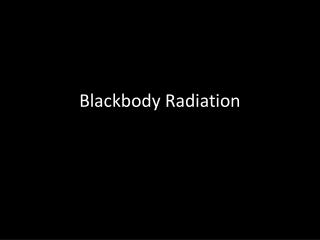DownloadDownload PresentationBlackbody Radiation

# Blackbody Radiation

Download Presentation## Blackbody Radiation

- - - - - - - - - - - - - - - - - - - - - - - - - - - E N D - - - - - - - - - - - - - - - - - - - - - - - - - - -
##### Presentation Transcript

1. Blackbody Radiation

2. Blackbody: an object that absorbs and emits all radiations of all possible frequencies • Kirchhoff observed that when heated to incandescence, gases emit certain frequencies of light, and that all objects absorb the same frequency of radiation they emit, making a blackbody a “perfect radiator”

3. The power radiated from a blackbody depends on the temperature • If the power of a blackbody doubles, the power emitted will increase by 2⌃4 times • It was believed that as the frequency increased so would the relative intensity. However, once the frequency passed into the ultraviolet spectrum, the relative intensity started decreasing. This is known as the ultraviolet catastrophe

4. Energy of an oscillator • E=nhf • E=energy (J) • n=positive interger (1,2,3…) • h=planck’s constant 6.63x10⌃-34 J*s • f=frequency of vibration (Hz)

5. There can be no discrete values of hf, any energy in between 1hf and 2hf, for example, does not exist. • An oscillator is an object that vibrates repetitively • Emissitivity: ratio of energy an object radiates compared to perfect radiation. The human body is beween 0.65 and 0.80

6. Law of radiation • Q=eσAtT⌃4 • Q=radiant energy (J) • e=emissitivity • σ=stefan-boltzman constant 5.7x10⌃-8 J/(m⌃2)(s)(k⌃4) • t=radiation time interval (s) • A=surface area (m) • T=temperature (k)

7. Questions • 1. What energy is emitted by an oscillator with a frequency of 2.3x10⌃5Hz? The discrete value is 4. • 2. What is the radiant energy of a very pasty human (e=0.65) who goes outside in 30 degree weather for 50 seconds, assuming the area exposed is 0.7m⌃2?

8. 3. If the energy emitted by an oscillator is 7.1x10⌃-31 J, and the positive integer is 2, what is the frequency? • 4. The radiant energy of an object is 80 J. If its emissitivity is 0.77, its surface area is 2 m⌃2, and the temperature is 298 K, how long was the object exposed?

9. http://www.youtube.com/watch?v=l_t8dn4c6_g